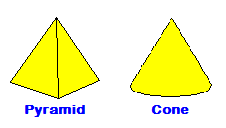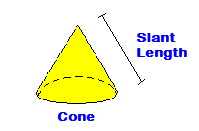# To The Point With Cone Math

Although the cone isn’t as popular, or as common as other shapes, it is still a mainstay in our daily lives.  Without it, there would be fewer options to hold your ice cream, it would be difficult to alter traffic around construction zones, and the funnel wouldn’t even exist.  How would we be able to pour fluids into a small space without a funnel?  Because of the unique qualities of this shape, it’s important to know the basic math behind the cone.

Let’s look at what a cone is.  It is a shape that is similar to a pyramid.  Instead of the pyramid having a square, or triangular base, it has a circle for a base.  The body extends from the circular base and meets at a point.The volume of a cone can be calculated from a combination of two equations.  First, we need to know the area of the circular base.  This equation is Pi x R2.  Pi is the constant estimated at 3.1416.  R is the radius of the circle.  Now that we have what we need to find the area of the cone base, we need to multiply that by 1/3 x Height to find the total volume.  This gives us a final equation for volume of 1/3 x H x Pi x R2.   Circle Area Calculator     Cone Volume Calculator

Another math function for cones is the surface area.  Again, this is a two part equation with the first part finding the area of the base.  Again, we use Pi x R2 for the area of the base, now we need to find the surface area of the body.  To find the body surface area we use Pi x R x S.  R is the radius of the base, and S is the length of the slant of the outside edge of the cone from the base to the point.  The slant can either be measured on it’s own, or you can use the Pythagorean theorem to find the slant length from the height and base radius.  The total combination of these two parts forms the equation for cone surface area. Pi x R2 x Pi x R x SNow that you have the basic math behind the geometry of cones, you can effectively find out how much ice cream is left in the bottom of your ice cream cone.  And we all know how important that is.

CalcuNATION is a website featuring online calculators and educational resources for mathematics.  Other Mathematical Blogs ( CalcuNATION on EduBlogs and CalcuNATION on Blogger)

To The Point With Cone Math
Scroll to top